# How to use the DECIMAL Function in Excel

In this article, we will learn about how to use the DECIMAL function in Excel.

DECIMAL function in excel is used to convert text representation of numbers of a given base to decimal numbers (base =10).

The table shown below shows you some of the most used base & their radix Alpha - numeric characters

 Base radix Alpha-Numeric Characters Binary 2 0 - 1 Octal 8 0 - 7 decimal 10 0 - 9 hexadecimal 16 0 - 9 & A - F hexatridecimal 36 0 - 9 & A - Z

The DECIMAL function converts the number of a given radix to a number of radix 10 (decimal).
Syntax:

=DECIMAL (number, radix)

Number : value to be converted to decimal.
Radix : or base of the given number. Range from 2 to 36 (integers).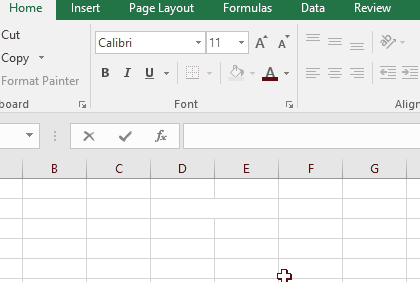The decimal value is obtained from the number of radix 2 as shown in the above gif.

Let’s understand this function using it in an example.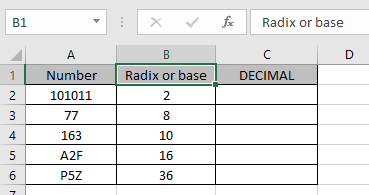The numbers & their Radix or base value. We need to convert these numbers to decimal value which is base 10.

Use the formula:

=DECIMAL (A2, B2)

A2 : number
B2 : radix or base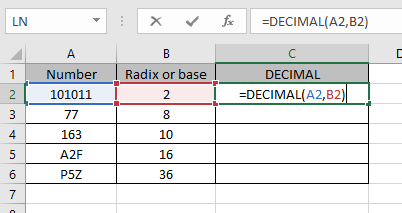Values to the DECIMAL function is provided as cell reference.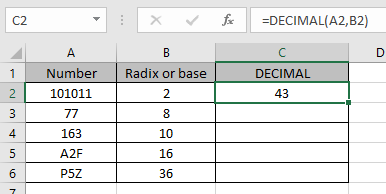The decimal representation of 101011 of base 2 is 43 of base 10.

Now copy the formula to other cells using the Ctrl + D shortcut key.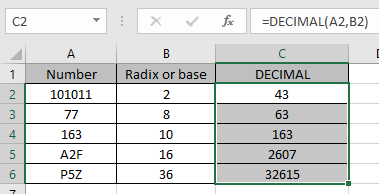As you can see here the decimal representation of the numbers from the numbers.
Notes:

1. The argument to the function can be given as text in quotes or cell reference. Numbers can be given as argument to the function directly without quotes or cell reference.
2. The number must be an Alpha numeric number to the given radix or base.
3. The function returns the #NUM! Error
• If radix is less than 2 or greater than 36.
• If the argument to the function is not readable by excel.
• The number characters exceed by 255.
4. The function returns the # VALUE! Error
• If the text argument exceeds the character limit of 255.
• If the radix integers is non - numeric.
5. Radix must be an integer greater than or equal to 2 and less than equal to 36.
6. Text argument to the function is not case sensitive.

Hope you understood how to use DECIMAL function and referring cell in Excel. Explore more articles on Excel mathematical conversion functions here. Please feel free to state your query or feedback for the above article.

Popular Articles:

50 Excel Shortcuts to Increase Your Productivity

How to use the VLOOKUP Function in Excel

How to use the COUNTIF in Excel 2016

How to use the SUMIF Function in Excel

Terms and Conditions of use

The applications/code on this site are distributed as is and without warranties or liability. In no event shall the owner of the copyrights, or the authors of the applications/code be liable for any loss of profit, any problems or any damage resulting from the use or evaluation of the applications/code.